Web www.handwritingforkids.com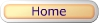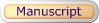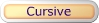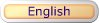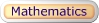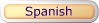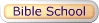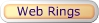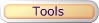### Division 4 - Dividing through Dividend

This printable basic math division worksheet generator will provides plenty of math practice, boost test scores and prepare the child/children to meet the Grade 3-5 curriculum standards. This math worksheet maker will create a horizontal division equations without handwriting lines. No Remainders

See sample math worksheets below:

dividend
divisor
quotient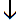4 ÷ 2 = 2

Follow these steps to make your worksheet.
1. Select the number of math problems per page. (Default: 1 - 18)
2. Select Type of Math Problems: (Default: Dividing through 81)
3. Click on submit to make your personalized horizontal math division worksheet.
4. Option: Page back and click Submit to create a different set of math problems.

Math - Division 4
Dividing through Dividend Worksheet Maker (Horizontal)

 Enter the Number of Math Problems per page: Select a range 1 - 3 1 - 6 1 - 9 1 - 12 1 - 15 1 - 18 Default: 1 - 18 Type of Math Problems: Select a Type of Math Dividing through 18 Dividing through 27 Dividing through 36 Dividing through 45 Dividing through 54 Dividing through 63 Dividing through 72 Dividing through 81 Default: Dividing through 81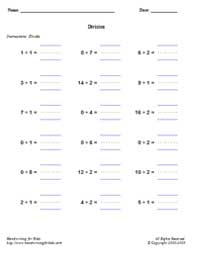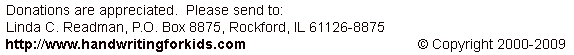Basic Handwriting for Kids Free for Non-Profit Use Ms. Readman, Rockford, IL Revised: